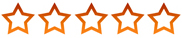# 2.2 Calculations in Warewolf

Watch this video to learn how to do calculations in Warewolf.

When you type the = sign in a right assign text field the  fx symbol appears. This means that you have now entered math mode.

Apart from standard mathematical functionality, Warewolf also has a powerful formula system built into it that is functionally and syntactically equivalent to Microsoft Excel.

There are 175 functions that cover the most commonly used calculations including: Compatibility, Date and time, Engineering, Financial, Information, Math and Trigonometry and Logical functions.

As an exercise, we are going to convert 6.5 pounds to kilograms. The syntax for the convert function is:

CONVERT (number, from_unit, to_unit)

The pound unit is “lbm”. The gram unit is “g”

1. Create a new workflow
2. Drag an Assign tool onto the design surface, and link it to the Start Node.
3. Create a new variable called [[PoundValue]] and set its value to 6.5
4. Create another variable called [[Answer]] Assign the following to it: (type this in) =(convert([[PoundValue]], “lbm”,”g”))/1000 as we want the value in kilograms not grams
5. Click Done

Press F6 to run your microservice in debug mode. The answer will be assigned to the [[Answer]] variable.

Now we are going to turn this microservice into a conversion service. To do this we simply need to:

• Clear the [[PoundValue]] variable, and the 6.5 value you assigned to it in the Assign tool on the design surface
• Flag [[PoundValue]] as an Input variable and [[Answer]] as an Output variable in the Variables box.
• To tidy it up a bit, let’s add KG to the end of [[Answer]]. To do this we add another variable in the Assign tool [[Answer]] = [[Answer]] KG.

And that’s it. We now have a microservice that converts pounds to kilograms. Click Debug from the toolbar to try it out.

Type a value into the PoundValue input field and click the View In Browser button.

You can change the input value simply by changing the assigned value of PoundValue in the URL without needing to press Debug each time.

Save your new microservice as PoundToKilogramConverter.

Updated on June 22, 2018